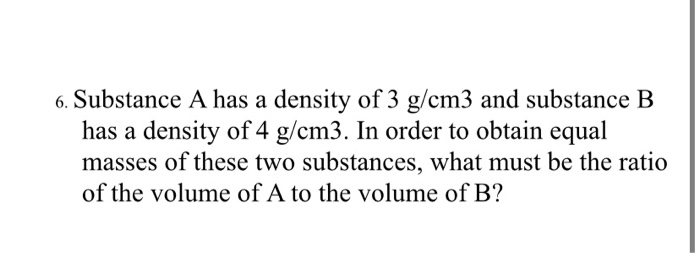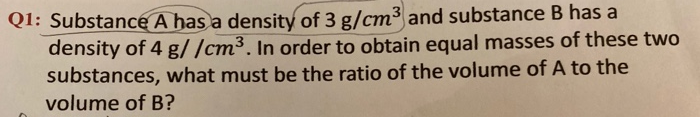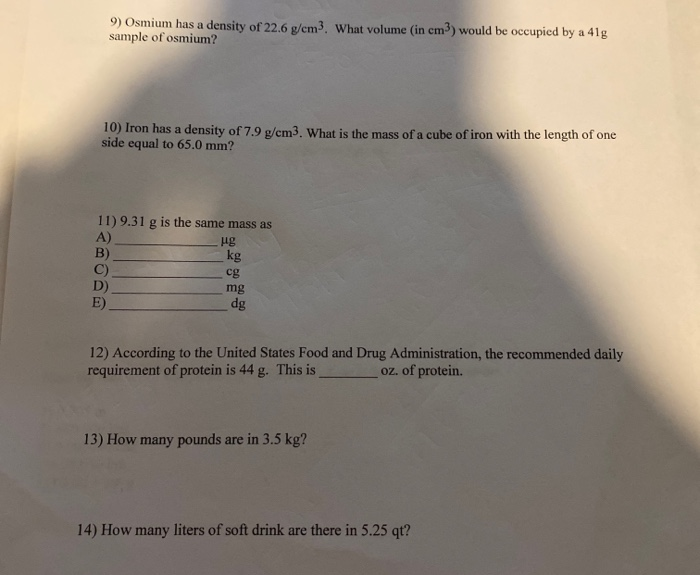# 6. Substance A has a density of 3 g/cm3 and substance B has a density of...6. Substance A has a density of 3 g/cm3 and substance B has a density of 4 g/cm3. In order to obtain equal masses of these two substances, what must be the ratio of the volume of A to the volume of B?

This Homework Help Question: "6. Substance A has a density of 3 g/cm3 and substance B has a density of..." No answers yet.

We need 10 more requests to produce the answer to this homework help question. Share with your friends to get the answer faster!

0 /10 have requested the answer to this homework help question.

Once 10 people have made a request, the answer to this question will be available in 1-2 days.
All students who have requested the answer will be notified once they are available.

#### Earn Coin

Coins can be redeemed for fabulous gifts.

Similar Homework Help Questions
• ### Q1: Substance A has a density of 3 g/cm3 and substance B has a density of...Q1: Substance A has a density of 3 g/cm3 and substance B has a density of 4 g//cm3. In order to obtain equal masses of these two substances, what must be the ratio of the volume of A to the volume of B?

• ### Suppose an unknown substance has a melting temperature of 1,200 degrees Celsius and a density of 16.7 g/cm3

Suppose an unknown substance has a melting temperature of 1,200 degrees Celsius and a density of 16.7 g/cm3. However, the different individual atoms that make up the element have very different melting temperatures and densities. The unknown substance is most likely a what?

• ### What volume of lead (of density 11.3 g/cm3) has the same mass as 304 cm3 of a piece of redwood (of density 0.38 g/cm3

What volume of lead (of density 11.3 g/cm3) has the same mass as 304 cm3 of a piece of redwood (of density 0.38 g/cm3?(Answer in units of cm3.Okay, so this is like testing us over the basics, but I really can't get the answer! I tried everything...

• ### Suppose an unknown substance has a melting temperature of 1,200 degrees Celsius and a density of 16.7 g/cm3

Suppose an unknown substance has a melting temperature of 1,200 degrees Celsius and a density of 16.7 g/cm3. However, the different individual atoms that make up the substance have very different melting temperatures and densities. The unknown substance is most likely a compound, solution, or a molecule

• ### Acetone has a density of 0.7857 g/cm3

Acetone has a density of 0.7857 g/cm3. What is the mass,in grams, of 28.56mL of acetone? What is the volume, in milliliters, of 6.54 g of acetone?

• ### Suppose an unknown substance has a melting temperature of 1,200 degrees Celsius and a density of 16.7 g/cm3

Suppose an unknown substance has a melting temperature of 1,200 degrees Celsius and a density of 16.7 g/cm3. However, the different individual atoms that make up the element have very different melting temperatures and densities. Which is the unknown substance most likely

• ### If 57.9 grams of gold has a volume of 3 cubic centimeters what is the density of gold in g/ cm3,

If 57.9 grams of gold has a volume of 3 cubic centimeters what is the density of gold in g/ cm3,?

• ### An ethanol-water solution is 40.00% ethanol by mass and has a density of 0.9450 (g/cm3) at 25.00°C. The density of pure...

An ethanol-water solution is 40.00% ethanol by mass and has a density of 0.9450 (g/cm3) at 25.00°C. The density of pure ethanol is 0.7850 (g/cm3) and the density of pure water is 0.9970(g/cm3) at this temperature. At these conditions, the partial molar volume of ethanol is 55.00 (cm3/mol) and the partial molar volume of water is 17.5 (cm3/mol). a. Calculate the total volume of a 1000.0 g solution assuming both ideal and non-ideal conditions. b. What does the difference between...

• ### 9) Osmium has a density of 22.6 g/cm3. What volume (in cm) would be occupied by...9) Osmium has a density of 22.6 g/cm3. What volume (in cm) would be occupied by a 41g sample of osmium? 10 Iron has a density of 7.9 g/cm3. What is the mass of a cube of iron with the length of one side equal to 65.0 mm? 11) 9.31 g is the same mass as A) ug B) kg C) D) mg E) dg се 12) According to the United States Food and Drug Administration, the recommended daily requirement...

• ### What is the volume of a piece of iron that has a density of 7.874 g/cm3...

What is the volume of a piece of iron that has a density of 7.874 g/cm3 and a mass of 10.0000 grams?